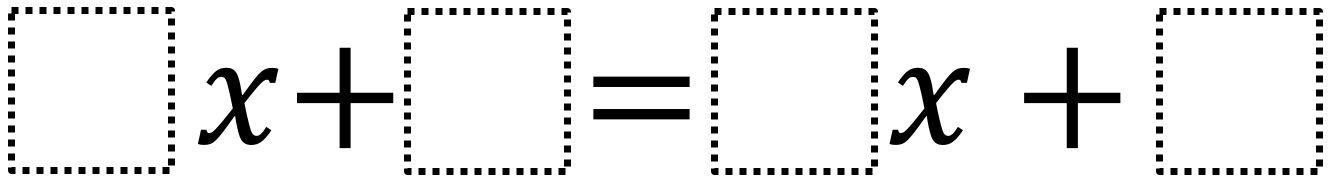# Solving Equations with Variables on Both Sides

Directions: Using the digits 1 to 9, at most TWO times each, fill in the boxes to make an equation with no solutions.### Hint

How can you tell when an equation has no solution?
How can you tell when an equation has infinite solutions?

### Answer

There are many answers, but the coefficient of both x terms have to be the same and the constants must have different values. So, 2x + 3 = 2x + 4 is an answer because it is equivalent to 3 = 4, for which there is no solution.

Source: Robert Kaplinsky

## Systems of Inequalities 2

Directions: Using the integers −9 to 9 at most one time each, place an integer …

### 80 comments

1.1x + 9 = 1x + 8
2x + 9 = 2x + 8
3x + 9 = 3x + 8
etc.

2.3.2x + 3 = 2x + 4

4.5x+10=5x+9

5.2x+3=2x+4

6.2x+3=2x+4.

7.2x+9=2x+2

8.9.1x+1=1x+2

10.11.4x+5=4x+4

12.sophia antogiovanni

5x+6=5x+5

13.Preston Shanosky

x+7=x+8

14.15.3x+7=3x+6

16.4x+8=4x+7

17.2x+5= 2x+4

18.3x+1=3x+6

19.4x+5=4x+6

20.7x+3=7x-4

21.2x + 3 = 2x + 4

22.23.7x + 4 = 7x + 3

24.2x+4=2x+9

25.5x+1=5x+4

26.9x+4=9x+8

27.3x+1=3x=4

28.29.•3x+9=3x+8

30.3x+9=3x+5

31.2x+1=2x+2

32.3x-5=3x+17

33.william hockstein

9x+3=9x+4

34.3x+7=3x+6

35.8x+5=8x+9

36.4x+5=4x+4

37.6x +7 = 6x + 2

38.9x+2= 9x+1

39.5x+3=5x-7

40.5x+3=5x+2

41.3x+2=3x+5

42.2x+1=2x+3

43.2x+7=2x+6

44.6x-5=6x+3

45.4x+5=4x+7

46.7x-2=7x+5

47.4x+8= 4x+2

48.486x+77=39x+45

49.50.51.5x+3=5x-6

52.4x+5=4x-6

53.1x+9=2x+4

54.55.56.8x+3=8x+5

57.58.x-3=x-2

59.2x + 3 = 2x + 8

60.4x-5=4x-6

61.2x+3=2×4

62.63.percydous Guerra

7x+3=2x+13

64.percydous Guerra

7x+3=2x=13

65.3x+7=3x+8

66.5x+7=5x+8

67.18x+4=18x+7

68.5x+7=5x+6

69.13x+9=13x+8

70.Solving Equations with Variables on Both Sides
Directions: Using the digits 1 to 9, at most TWO times each, fill in the boxes to make an equation with no solutions.
_x +_ =_ x + _ -> original problem
2x +5 = 2x +9 -> my attempt

71.72.2x+4=2x+3

73.2x+4=2x+4

74.2x+4=2x+3

75.4x+5=4x+6

76.To keep in line with the structure of other open middle problems, perhaps you could change it to

Directions: Using the digits 1 to 9, at most ONE time each, fill in the boxes to make an equation with no solutions.

__ x + ___ = ___ ( ___ x + ___ )

or

__ x + ___ = ___ x + ___ – ___ x

77.78.3x+ 6= 5x +4

79.-11x=4=-11x+-11